# SimBiology.gsa.ElementaryEffects

Object containing results from calculation of elementary effects for global sensitivity analysis (GSA)

## Description

The `SimBiology.gsa.ElementaryEffects` object contains GSA results returned by `sbioelementaryeffects`. The object contains the computed elementary effects with respect to parameter inputs.

## Creation

Create a `SimBiology.gsa.ElementaryEffects` object using `sbioelementaryeffects`.

## Properties

expand all

Flag to use the absolute values of elementary effects, specified as `true` or `false`. By default, the function uses the absolute values of elementary effects. Using nonabsolute values can average out when calculating the mean. For details, see Elementary Effects for Global Sensitivity Analysis.

Data Types: `logical`

Time points at which elementary effects are computed, specified as a column numeric vector. The property is `[]` if all observables are scalars.

Data Types: `double`

GSA results with elementary effects, specified as a structure array. The size of the array is params-by-observables, where params is the number of input parameters (sensitivity inputs) and observables is the number of observables (sensitivity outputs).

Each structure contains the following fields.

• `Parameter` — Name of an input parameter, specified as a character vector

• `Observable` — Name of an observable, specified as a character vector

• `Mean` — Mean of absolute values of elementary effects, specified as a scalar or numeric vector

• `StandardDeviation` — Standard deviation of absolute elementary effects, specified as a scalar or numeric vector

If all observables are scalar, then the `Mean` and `StandardDeviation` fields are specified as scalars. If any observables are vectors, `Mean` and `StandardDeviation` are numeric vectors of length `Time`. If some observables are scalars and some are vectors, scalar observables are scalar-expanded, where each time point has the same value.

Data Types: `struct`

Discretization level of the parameter domain, specified as a positive even integer. This parameter defines a grid of equidistant points in the parameter domain, where each dimension is discretized using `Gridlevel+1` points. The following figure shows an example of a grid for parameters p1 and p2 within given parameter bounds.For details, see Elementary Effects for Global Sensitivity Analysis.

Data Types: `double`

Step size for computing elementary effects, specified as a positive integer between 1 and `GridLevel`. The step size is measured in terms of grid points between neighboring points. The following figure shows examples of different grid delta values.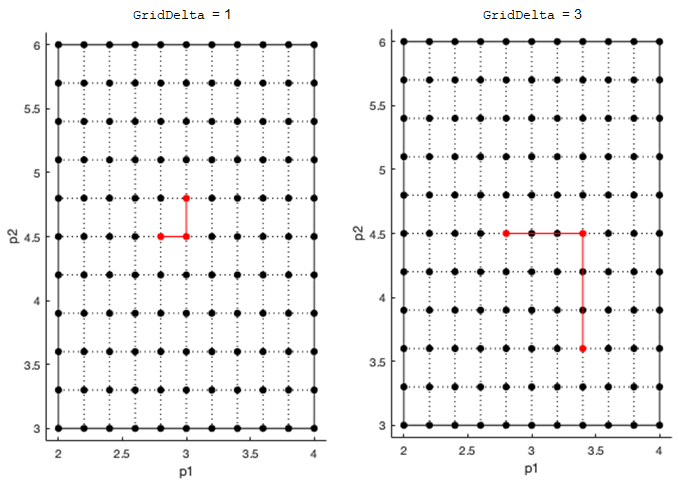For details, see Elementary Effects for Global Sensitivity Analysis.

Data Types: `double`

Method to select sample points to compute elementary effects, specified as `"chain"` or `"radial"`. The `"chain"` point selection uses the Morris method . The `"radial"` point selection uses the Sohier method . For details, see Elementary Effects for Global Sensitivity Analysis.

Data Types: `double`

Sampled parameter values, specified as a table. The table contains `(1+numel(params))*NumberSamples` rows and `numel(params)` columns.

`sbioelementaryeffects` uses blocks of `k+1` rows, where k is the number of input params, to compute an elementary effect for each input parameter. The total number of these blocks is equal to the total number of samples. You can get the block indices by running `kron((1:NumberSamples)',ones(numel(params)+1,1))`. For details, see Elementary Effects for Global Sensitivity Analysis.

Data Types: `table`

Names of model responses or observables, specified as a cell array of character vectors.

Data Types: `char`

Simulation information, such as simulation data and parameter samples, used for computing elementary effects, specified as a structure. The structure contains the following fields.

• `SimFunction``SimFunction` object used for simulating model responses or observables.

• `SimData``SimData` array of size `[NumberSamples,1]`, where NumberSamples is the number of samples. The array contains simulation results from `ParameterSamples`.

• `OutputTimes` — Numeric column vector containing the common time vector of all `SimData` objects.

• `Bounds` — Numeric matrix of size `[params,2]`. params is the number of input parameters. The first column contains the lower bounds and the second column contains the upper bounds for sensitivity inputs.

• `DoseTables` — Cell array of dose tables used for the `SimFunction` evaluation. `DoseTables` is the output of `getTable(doseInput)`, where doseInput is the value specified for the `'Doses'` name-value pair argument in the call to `sbiosobol`, `sbiompgsa`, or `sbioelementaryeffects`. If no doses are applied, this field is set to `[]`.

• `ValidSample` — Logical vector of size `[NumberSamples,1]` indicating whether a simulation for a particular sample failed. Resampling of the simulation data (`SimData`) can result in `NaN` values if the data is extrapolated. Such `SimData` are indicated as invalid.

• `InterpolationMethod` — Name of the interpolation method for `SimData`.

• `SamplingMethod` — Name of the sampling method used to draw `ParameterSamples`.

• `RandomState` — Structure containing the state of `rng` before drawing `ParameterSamples`.

Data Types: `struct`

## Object Functions

 `resample` Resample Sobol indices or elementary effects to new time vector `addobservable` Compute Sobol indices or elementary effects for new observable expression `removeobservable` Remove Sobol indices or elementary effects of observables `addsamples` Add additional samples to increase accuracy of Sobol indices or elementary effects analysis `plotData` Plot quantile summary of model simulations from global sensitivity analysis (requires Statistics and Machine Learning Toolbox) `plot` Plot means and standard deviations of elementary effects `bar` Plot magnitudes of means and standard deviations of elementary effects `plotGrid` Plot parameter grid and points used to compute elementary effects

## Examples

collapse all

`sbioloadproject tumor_growth_vpop_sa.sbproj`

Get a variant with estimated parameters and the dose to apply to the model.

```v = getvariant(m1); d = getdose(m1,'interval_dose');```

Get the active configset and set the tumor weight as the response.

```cs = getconfigset(m1); cs.RuntimeOptions.StatesToLog = 'tumor_weight';```

Simulate the model and plot the tumor growth profile.

`sbioplot(sbiosimulate(m1,cs,v,d));`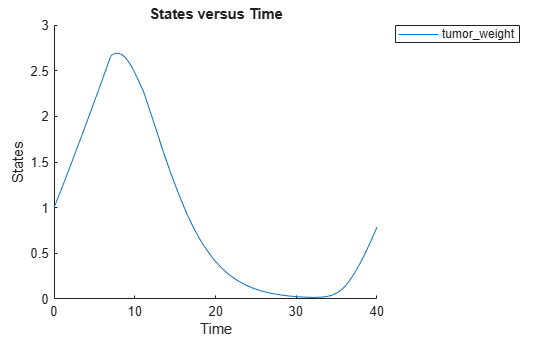Perform global sensitivity analysis (GSA) on the model to find the model parameters that the tumor growth is sensitive to.

First, define model parameters of interest, which are involved in the pharmacodynamics of the tumor growth. Define the model response as the tumor weight.

```modelParamNames = {'L0','L1','w0','k1'}; outputName = 'tumor_weight';```

Then perform GSA by computing the elementary effects using `sbioelementaryeffects`. Use `100` as the number of samples and set `ShowWaitBar` to `true` to show the simulation progress.

```rng('default'); eeResults = sbioelementaryeffects(m1,modelParamNames,outputName,Variants=v,Doses=d,NumberSamples=100,ShowWaitbar=true);```

Show the median model response, the simulation results, and a shaded region covering 90% of the simulation results.

`plotData(eeResults,ShowMedian=true,ShowMean=false);`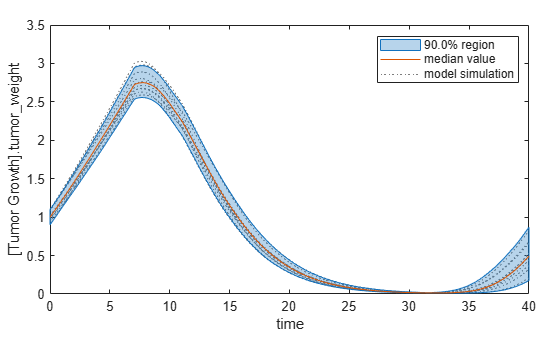You can adjust the quantile region to a different percentage by specifying `Alphas `for the lower and upper quantiles of all model responses. For instance, an `alpha` value of 0.1 plots a shaded region between the `100*alpha` and `100*(1-alpha)` quantiles of all simulated model responses.

`plotData(eeResults,Alphas=0.1,ShowMedian=true,ShowMean=false);`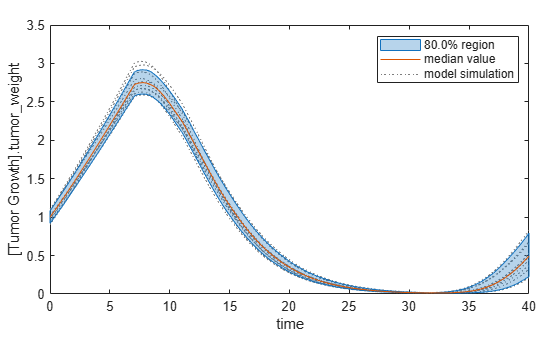Plot the time course of the means and standard deviations of the elementary effects.

```h = plot(eeResults); % Resize the figure. h.Position(:) = [100 100 1280 800];```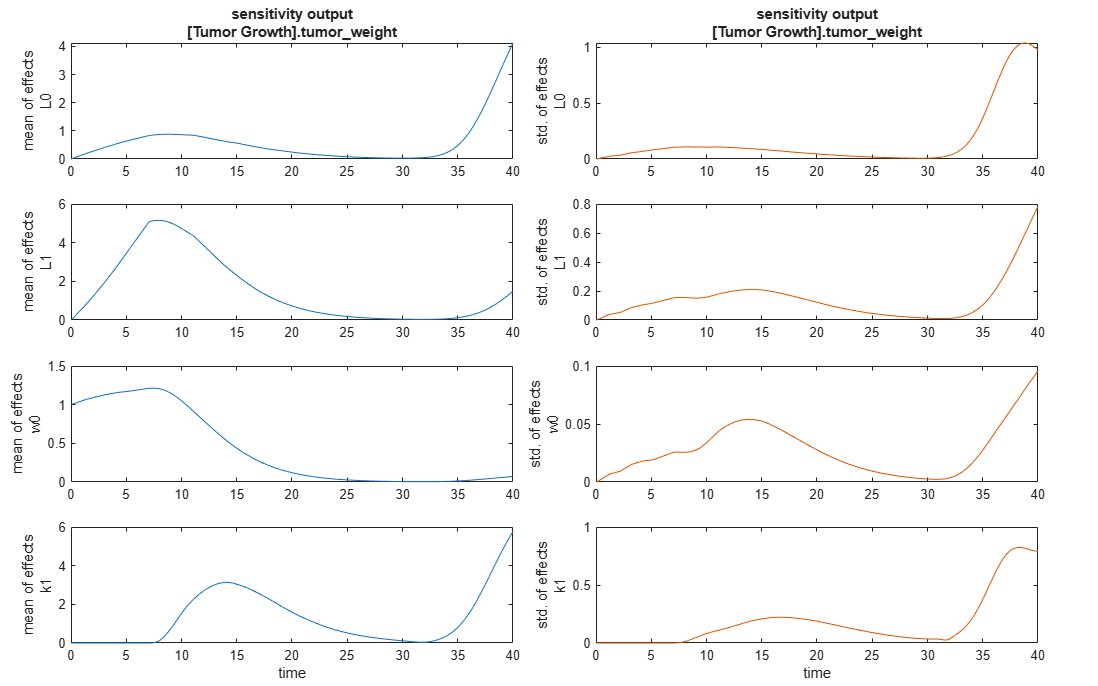The mean of effects explains whether variations in input parameter values have any effect on the tumor weight response. The standard deviation of effects explains whether the sensitivity change is dependent on the location in the parameter domain.

From the mean of effects plots, parameters `L1` and `w0` seem to be the most sensitive parameters to the tumor weight before the dose is applied at t = 7. But, after the dose is applied, `k1` and `L0` become more sensitive parameters and contribute most to the after-dosing stage of the tumor weight. The plots of standard deviation of effects show more deviations for the larger parameter values in the later stage (t > 35) than for the before-dose stage of the tumor growth.

You can also display the magnitudes of the sensitivities in a bar plot. Each color shading represents a histogram representing values at different times. Darker colors mean that those values occur more often over the whole time course.

`bar(eeResults);`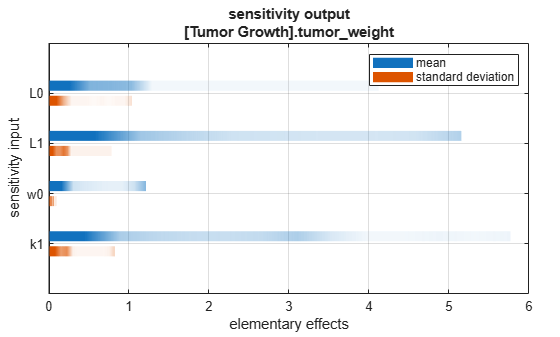You can also plot the parameter grids and samples used to compute the elementary effects.

`plotGrid(eeResults)`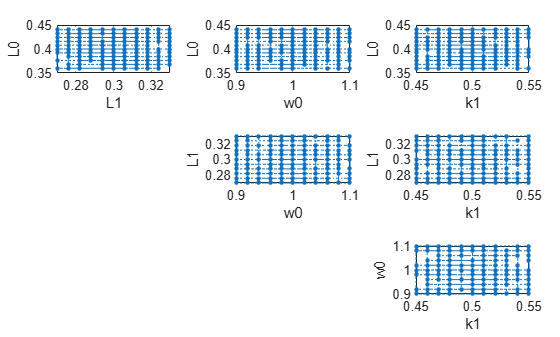You can specify more samples to increase the accuracy of the elementary effects, but the simulation can take longer to finish. Use `addsamples` to add more samples.

`eeResults2 = addsamples(eeResults,200);`

The `SimulationInfo` property of the result object contains various information for computing the elementary effects. For instance, the model simulation data (SimData) for each simulation using a set of parameter samples is stored in the `SimData` field of the property. This field is an array of `SimData` objects.

`eeResults2.SimulationInfo.SimData`
``` SimBiology SimData Array : 1500-by-1 Index: Name: ModelName: DataCount: 1 - Tumor Growth Model 1 2 - Tumor Growth Model 1 3 - Tumor Growth Model 1 ... 1500 - Tumor Growth Model 1 ```

You can find out if any model simulation failed during the computation by checking the `ValidSample` field of `SimulationInfo`. In this example, the field shows no failed simulation runs.

`all(eeResults2.SimulationInfo.ValidSample)`
```ans = logical 1 ```

You can add custom expressions as observables and compute the elementary effects of the added observables. For example, you can compute the effects for the maximum tumor weight by defining a custom expression as follows.

```% Suppress an information warning that is issued. warnSettings = warning('off', 'SimBiology:sbservices:SB_DIMANALYSISNOTDONE_MATLABFCN_UCON'); % Add the observable expression. eeObs = addobservable(eeResults2,'Maximum tumor_weight','max(tumor_weight)','Units','gram');```

Plot the computed simulation results showing the 90% quantile region.

```h2 = plotData(eeObs,ShowMedian=true,ShowMean=false); h2.Position(:) = [100 100 1500 800];```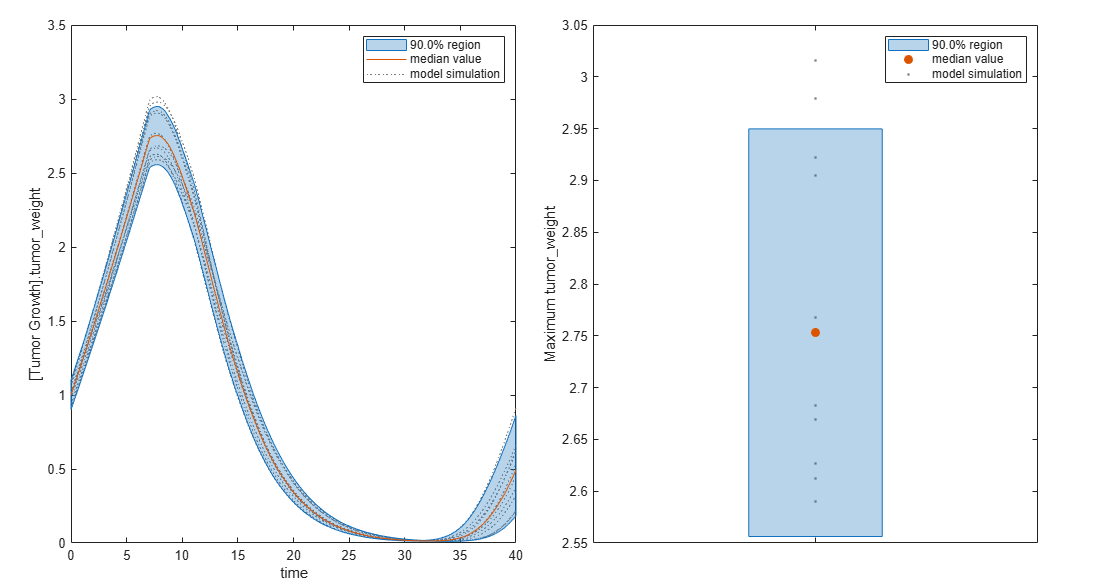You can also remove the observable by specifying its name.

`eeNoObs = removeobservable(eeObs,'Maximum tumor_weight');`

Restore the warning settings.

`warning(warnSettings);`

 Morris, Max D. “Factorial Sampling Plans for Preliminary Computational Experiments.” Technometrics 33, no. 2 (May 1991): 161–74.

 Sohier, Henri, Jean-Loup Farges, and Helene Piet-Lahanier. “Improvement of the Representativity of the Morris Method for Air-Launch-to-Orbit Separation.” IFAC Proceedings Volumes 47, no. 3 (2014): 7954–59.